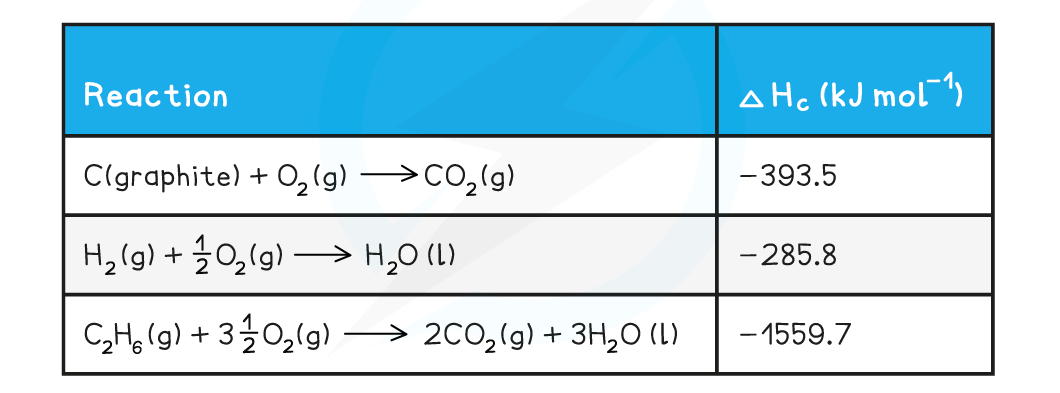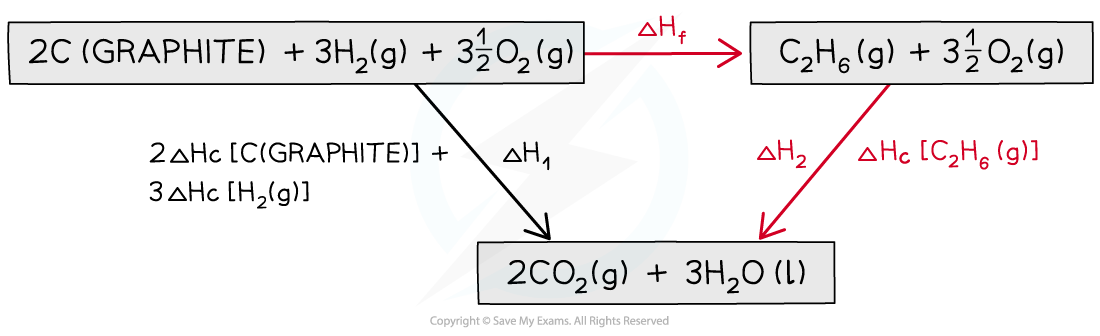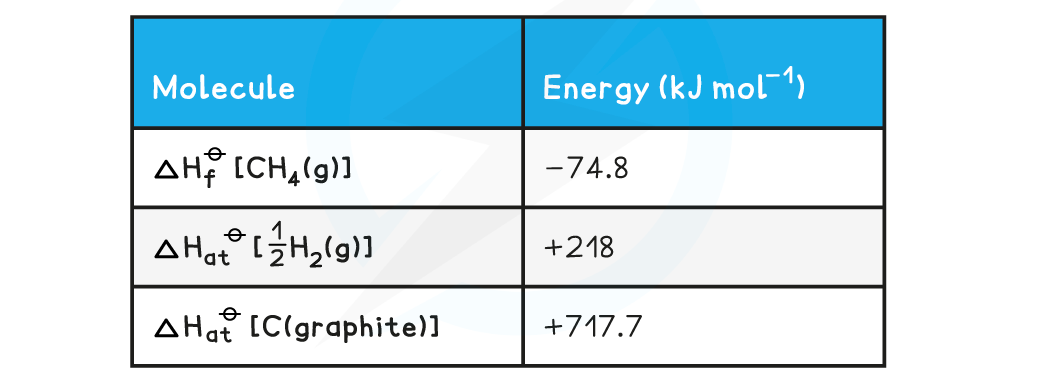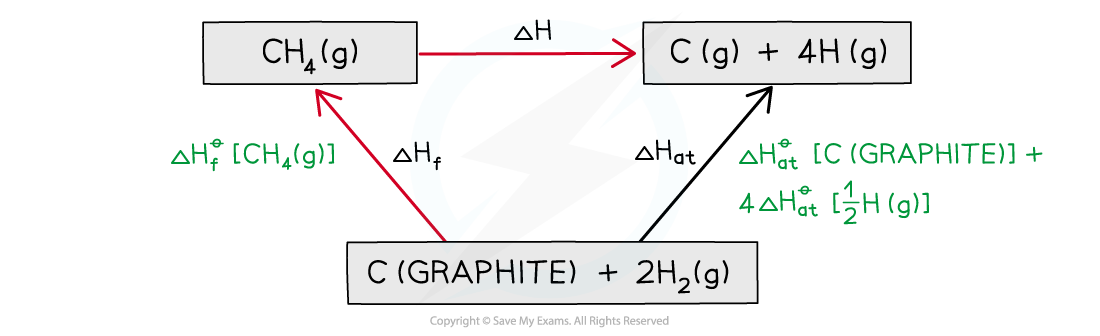# AQA A Level Chemistry复习笔记1.6.6 Applications of Hess’s Law

### Hess' Law Calculations

• You must make sure that you can apply Hess' Law effectively and calculate enthalpy changes in different situations
• Remember - it is the data that is important
• Check whether the data you have been given is formation data or combustion data, and then complete the cycle or calculation according to that

#### Worked Example

Calculating the enthalpy change of formation of ethaneCalculate ΔHf [ethane]. The relevant change in standard enthalpy of combustion (ΔHc) values are shown in the table below:Step 1: Write the equation for enthalpy change of formation at the top and add oxygen on both sidesStep 2: Draw the cycle with the combustion products at the bottomStep 3: Draw all arrows in the correct directionStep 4: Apply Hess’s Law#### Calculating average bond energies using Hess’s cycles

• Bond energies cannot be found directly so enthalpy cycles are used to find the average bond energy
• This can be done using enthalpy changes of atomisation and combustion or formation
• The enthalpy change of atomisation (ΔHatꝋ ) is the enthalpy change when one mole of gaseous atoms is formed from its elements under standard conditions.
• Eg. ΔHatꝋ [H2] relates to the equation:

½ H2(g) → H(g)

#### Worked Example

Calculating average C-H bond energyCalculate the average bond energy of the C-H bond using the relevant ΔHfꝋ and ΔHatꝋ values in the table below:Step 1: Write down the equation for the dissociation of methane at the topStep 2: Write down the elements at the bottomStep 3: Draw all arrows in the correct directionStep 4: Apply Hess’s LawStep 5: Since there are 4 C-H bonds in methane:#### Exam Tip

Remember to take into account the number of moles of each reactant and product.For example, there are two moles of NaHCO3(s) so the ΔHf value is multiplied by 2.